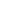# Mathematics for Computer Technology, 3e

By Robert McCullough  •
2006
•  480
Pages
Perfect bound
Format •
Print ISBN  9780895827005Suggested Student Retail Price

\$115.09

PurchaseRequest review copy

This book is designed for a one- (or possibly two-) semester course in mathematics for those students who are interested in computer programming. Although there will be numerous references to computers throughout the book, it is designed to be a mathematics text.

When talking about computer programming throughout the book, we will use a form of pseudo code, writing statements that roughly correspond to actual programming statements. This should make it easy for a student to change the pseudo code to whatever particular language is being used, if any. No prior programming knowledge is necessary.

Features
Instructor Resources
Features

In addition to many minor changes from the second edition, the following major changes have been made.

Throughout the book, references to specific programming languages, most notably BASIC, have been replaced by a form of pseudo code, which should make it easy for a student to convert from this pseudo code to whatever computer programming language he/she is using.

The set of integers will be designated by the letter Z, instead of the letter I, to make it more in line with current usage and to avoid confusion with the identity matrix.

In Chapter 1, the topics of order of operations and polynomials (Section 1.4) have been expanded upon and split into two sections.

In Chapter 2, the topics of domain and range of a function have been covered in more detail.

In Chapter 4, an additional method for finding the vertex of a parabola has been added.

In Chapter 6, the topic of complement addition (Sections 6.3-6.5) has been moved to Chapter 7. Two new sections (6.3 and 6.4) on subtraction in the various number systems have been added. Mixed operations in the binary number system have been added, as well as multiplication and division by powers of the base.

In Chapter 7, there have been numerous changes. The topics of engineering notation and metric notation have been added to the section on scientific notation (Section 7.2). Section 7.3 has been rewritten into two sections. The storage of integers using one byte for storage and addition and subtraction (by complement addition) has been added, as well as an expanded discussion of the problem of overflow. The storage of real numbers has been changed to comply with the IEEE standard for a single-precision number (32 bits). The ASCII code has been updated.

In Chapter 10, a section on Karnaugh maps (Section 10.6) has been added.

In Chapter 11, the examples and flowcharts have been rewritten using the pseudo code notation.

In Chapter 12, the dimensions of a matrix have been replaced by the size of a matrix to avoid confusion with multi-dimensional arrays.

In Chapter 14, Section 14.2, Introduction to Probability, has been rewritten.

Instructor Ancillaries: Login to view Instrucor Resources

• The Instructor's Manual contains answers to all questions.
Preface
Chapter 1: Basic Algebra
Chapter 2: Functions and Linear Equations
Chapter 3: Systems of Linear Equations
Chapter 4: Nonlinear Functions
Chapter 5: Number Systems
Chapter 6: Arithmetic in Different Number Systems
Chapter 7: Computer Considerations
Chapter 8: Sets
Chapter 9: Logic
Chapter 10: Boolean Algebra
Chapter 11: Computer Logic and Structured Programming
Chapter 12: Arrays and Matrices
Chapter 13: Linear Programming
Chapter 14: Probability and Statistics